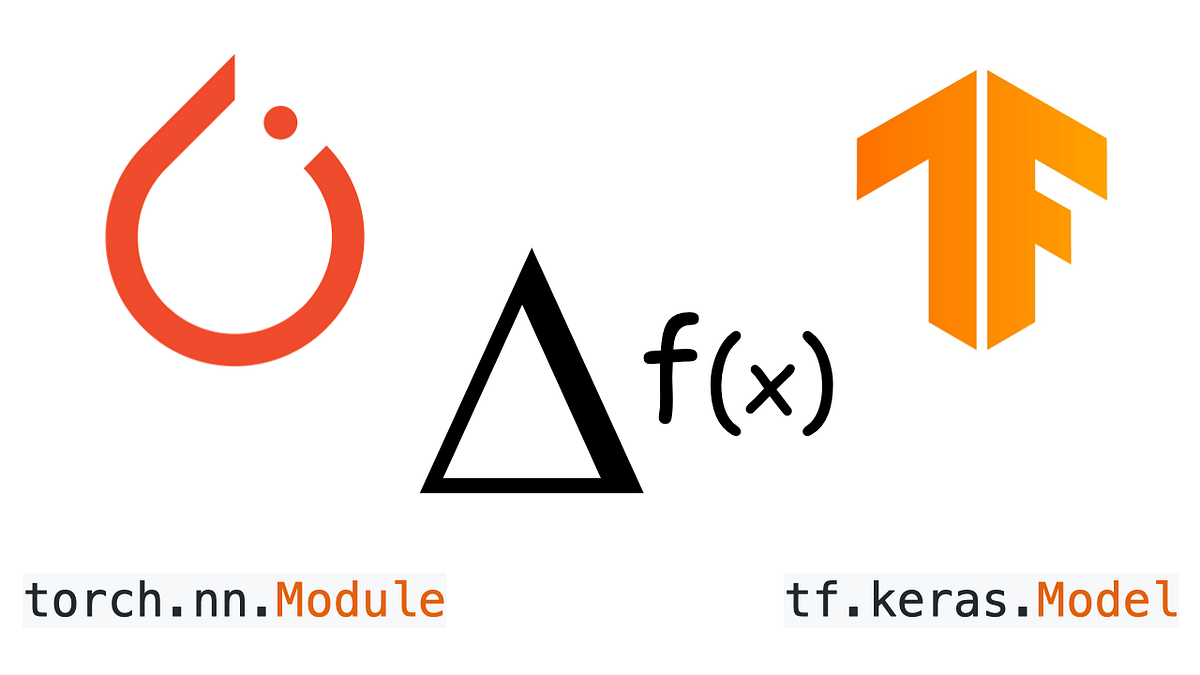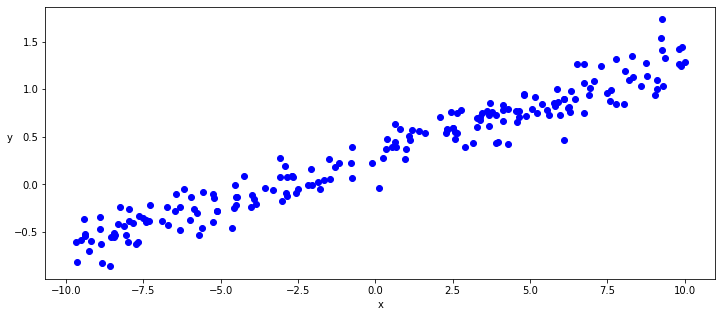# AutoDiff and Dynamic Subclassed Models with PyTorch Vs TensorFlowThis short article focus on how to use dynamic subclassed models with Module/Model API respectively in PyTorch 1.x and TensorFlow 2.x and how AutoDiff is used by these frameworks in the training loop to get the gradients of the loss.

This short article focus on how to use dynamic subclassed models with Module/Model API respectively in PyTorch 1.x and TensorFlow 2.x and how AutoDiff is used by these frameworks in the training loop to get the gradients of the loss and implement from scratch a very naive gradient descendant implementation.

## Generate some linear data with a bit of noise

With this intent of focusing on the core of auto-diff / auto-grad functionality we will use the simplest possible model, a linear regression model, and we will generate first, using numpy, some linear data adding a random level of noise.

``````def generate_data(m=0.1, b=0.3, n=200):
x = np.random.uniform(-10, 10, n)
noise = np.random.normal(0, 0.15, n)
y = (m * x + b ) + noise
return x.astype(np.float32), y.astype(np.float32)
x, y = generate_data()
plt.figure(figsize = (12,5))
ax = plt.subplot(111)
ax.scatter(x,y, c = "b", label="samples")``````## How Does It Stack Up Against Autograd, TensorFlow, and PyTorch?

In this article, take a look at accelerated automatic differentiation with Jax and see how it stacks up against Autograd, TensorFlow, and PyTorch.

## Pytorch vs Tensorflow in 2020

Pytorch and Tensorflow are by far two of the most popular frameworks for Deep Learning. It’s always a lot of work to learn and be comfortable with a new framework, so a lot of people face the dilemma of which one to choose out of the two

## My Journey in Converting PyTorch to TensorFlow Lite

My Journey in Converting PyTorch to TensorFlow Lite. Sometimes an MLOps gotta do what an MLOps gotta do

## Image Classification Model in PyTorch and TensorFlow

Understanding the Problem Statement: MNIST. In this post, you'll see Image Classification Model in PyTorch and TensorFlow

## PyTorch vs Tensorflow: Key Differences You Need To Know

PyTorch vs Tensorflow: Key Differences You Need To Know. This comparison blog on TensorFlow vs PyTorch provides you with a crisp knowledge about the top deep learning frameworks.